Coulombs Law and the Electric Field Physics 2415

• Slides: 22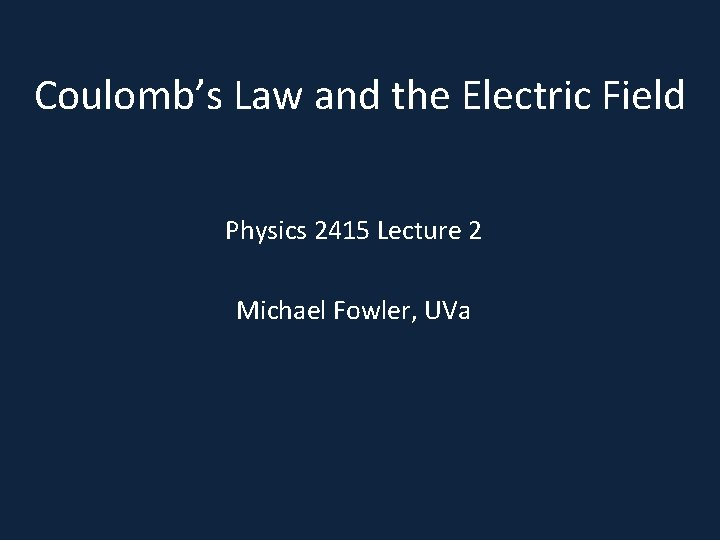Coulomb’s Law and the Electric Field Physics 2415 Lecture 2 Michael Fowler, UVa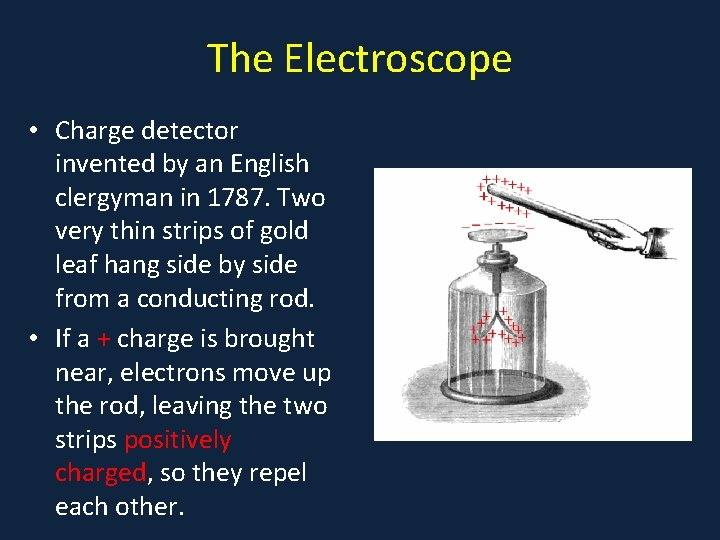The Electroscope • Charge detector invented by an English clergyman in 1787. Two very thin strips of gold leaf hang side by side from a conducting rod. • If a + charge is brought near, electrons move up the rod, leaving the two strips positively charged, so they repel each other.Charging the Electroscope… • By conduction: touch the top conductor with a positively charged object—this will leave it positively charged (electron deficient). • By induction: while holding a positively charged object near, but not in contact, with the top, you touch the electroscope: negative charge will flow from the ground, through you, to the electroscope.Coulomb’s Law • Coulomb measured the electrical force between charged spheres with apparatus exactly like Cavendish’s measurement of G: two spheres, like a dumbbell, suspended by a thin wire. One sphere was charged, another charged sphere was brought close, the angle of twist of the wire measured the force.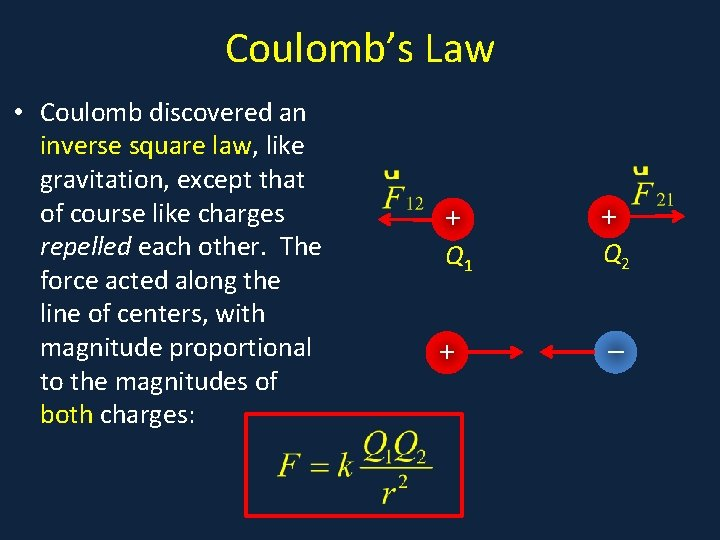Coulomb’s Law • Coulomb discovered an inverse square law, like gravitation, except that of course like charges repelled each other. The force acted along the line of centers, with magnitude proportional to the magnitudes of both charges: • a + Q 1 + + Q 2 _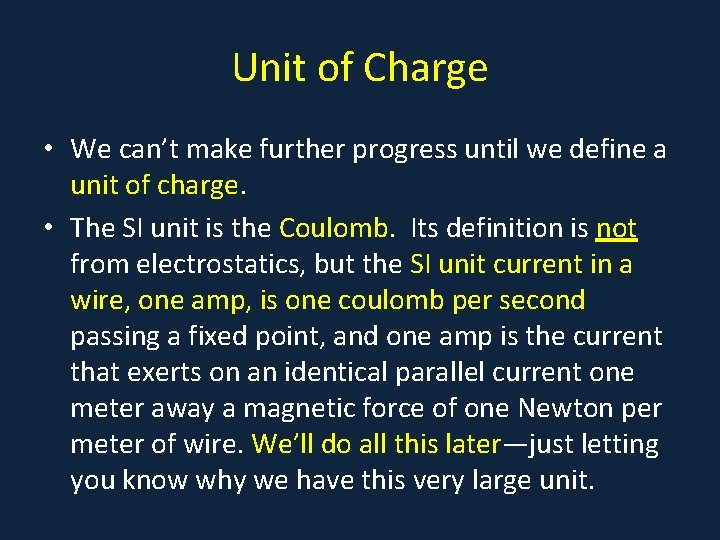Unit of Charge • We can’t make further progress until we define a unit of charge. • The SI unit is the Coulomb. Its definition is not from electrostatics, but the SI unit current in a wire, one amp, is one coulomb per second passing a fixed point, and one amp is the current that exerts on an identical parallel current one meter away a magnetic force of one Newton per meter of wire. We’ll do all this later—just letting you know why we have this very large unit.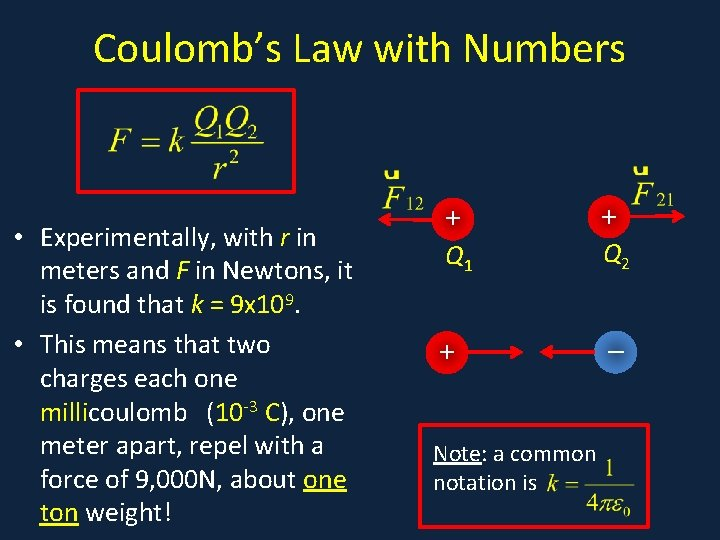Coulomb’s Law with Numbers • a • Experimentally, with r in meters and F in Newtons, it is found that k = 9 x 109. • This means that two charges each one millicoulomb (10 -3 C), one meter apart, repel with a force of 9, 000 N, about one ton weight! + Q 1 + Note: a common notation is + Q 2 _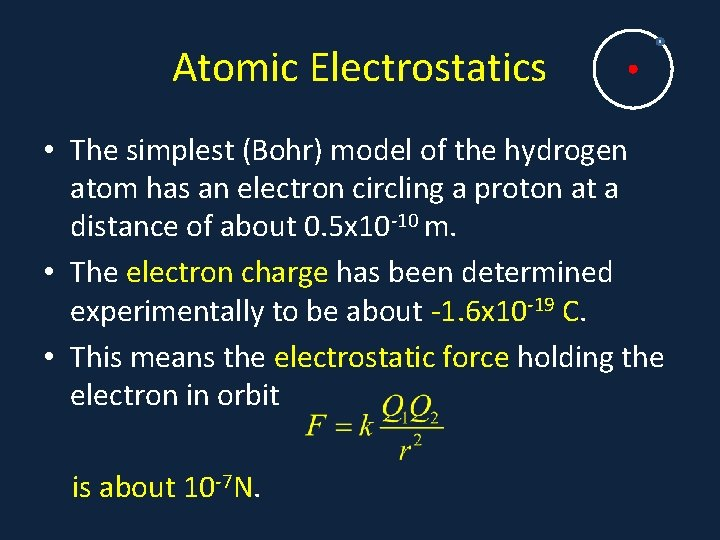Atomic Electrostatics • The simplest (Bohr) model of the hydrogen atom has an electron circling a proton at a distance of about 0. 5 x 10 -10 m. • The electron charge has been determined experimentally to be about -1. 6 x 10 -19 C. • This means the electrostatic force holding the electron in orbit is about 10 -7 N.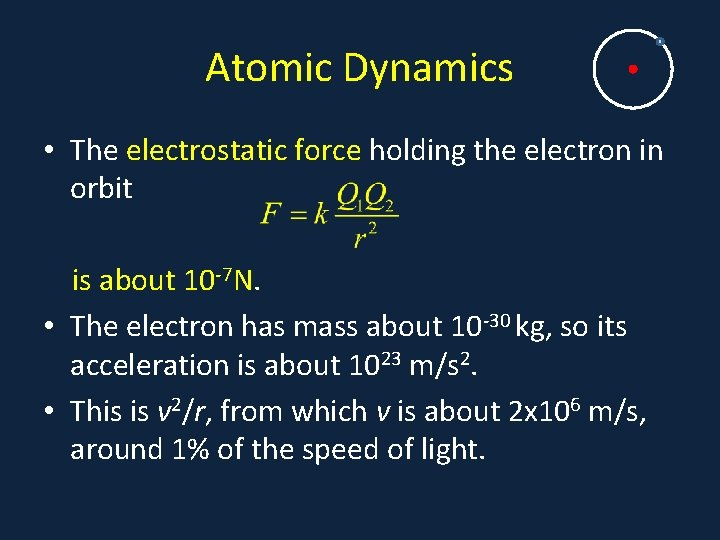Atomic Dynamics • The electrostatic force holding the electron in orbit is about 10 -7 N. • The electron has mass about 10 -30 kg, so its acceleration is about 1023 m/s 2. • This is v 2/r, from which v is about 2 x 106 m/s, around 1% of the speed of light.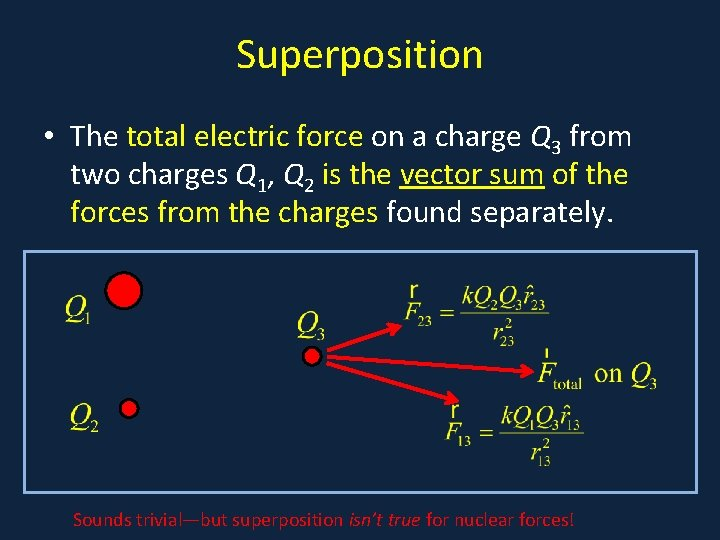Superposition • The total electric force on a charge Q 3 from two charges Q 1, Q 2 is the vector sum of the forces from the charges found separately. Sounds trivial—but superposition isn’t true for nuclear forces!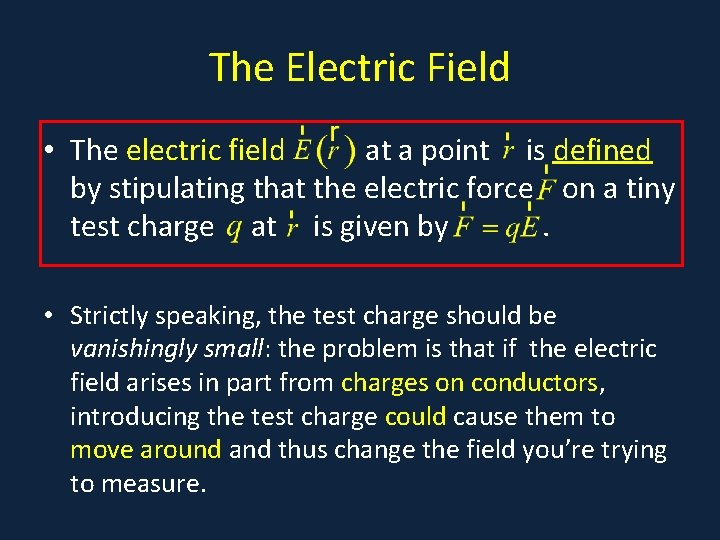The Electric Field • The electric field at a point is defined by stipulating that the electric force on a tiny test charge at is given by. • Strictly speaking, the test charge should be vanishingly small: the problem is that if the electric field arises in part from charges on conductors, introducing the test charge could cause them to move around and thus change the field you’re trying to measure.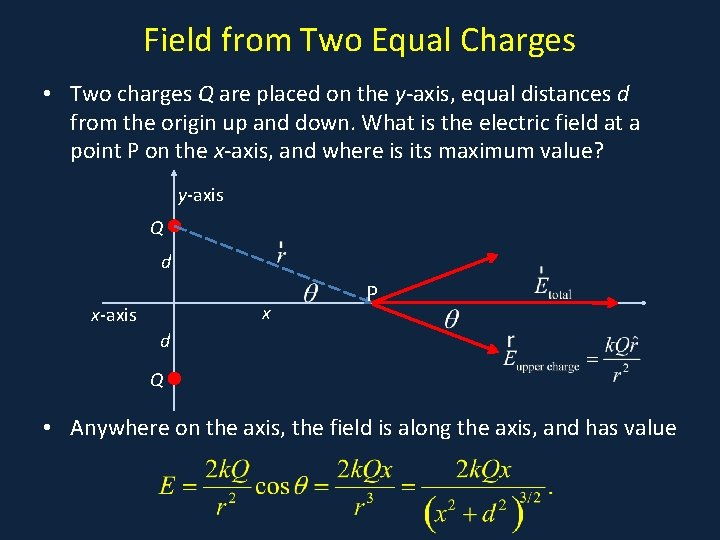Field from Two Equal Charges • Two charges Q are placed on the y-axis, equal distances d from the origin up and down. What is the electric field at a point P on the x-axis, and where is its maximum value? y-axis Q d x x-axis P d Q • Anywhere on the axis, the field is along the axis, and has value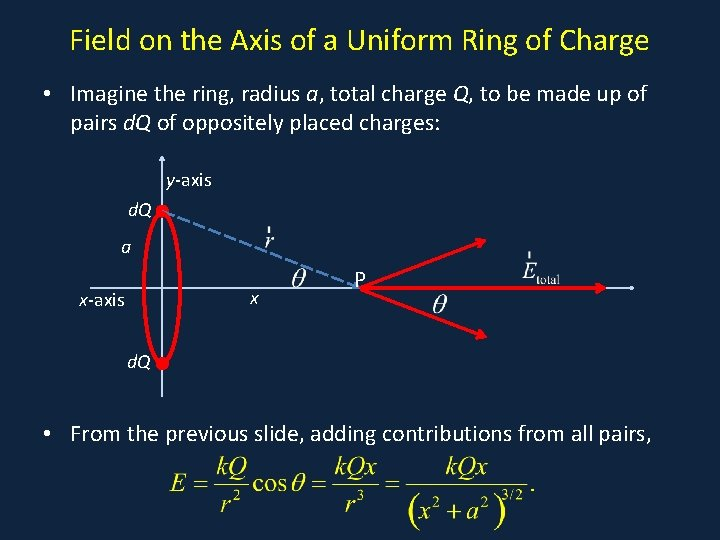Field on the Axis of a Uniform Ring of Charge • Imagine the ring, radius a, total charge Q, to be made up of pairs d. Q of oppositely placed charges: y-axis d. Q a x x-axis P d. Q • From the previous slide, adding contributions from all pairs,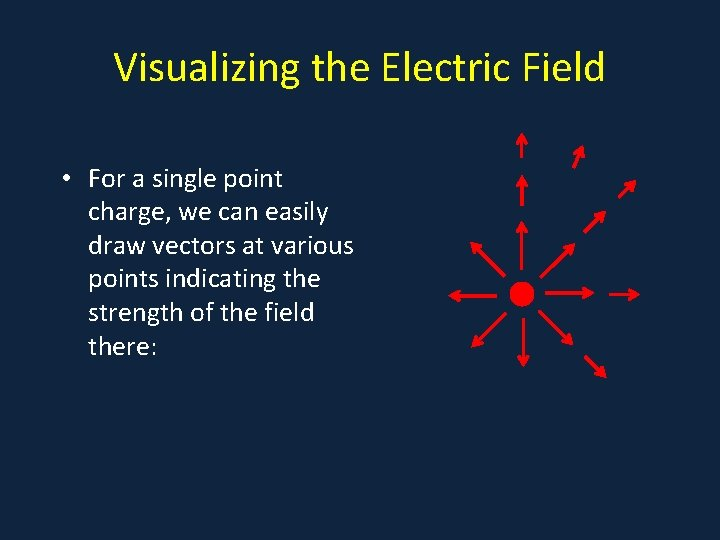Visualizing the Electric Field • For a single point charge, we can easily draw vectors at various points indicating the strength of the field there: • aVisualizing the Electric Field • A standard approach is to • a draw lines of force: lines that at every point indicate the field direction there. • These lines do not immediately give the field strength, but their density can give a qualitative indication of where the field is stronger, provided they are continuous.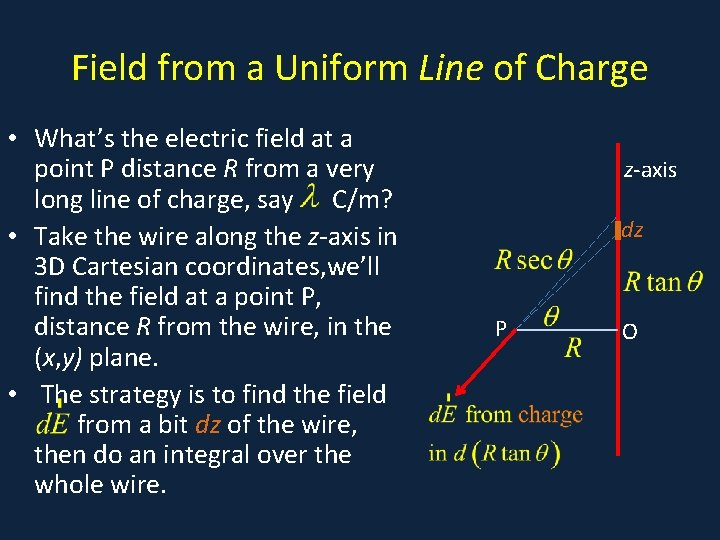Field from a Uniform Line of Charge • What’s the electric field at a • q point P distance R from a very long line of charge, say C/m? • Take the wire along the z-axis in 3 D Cartesian coordinates, we’ll find the field at a point P, distance R from the wire, in the (x, y) plane. • The strategy is to find the field z from a bit dz of the wire, then do an integral over the whole wire. z-axis dz P O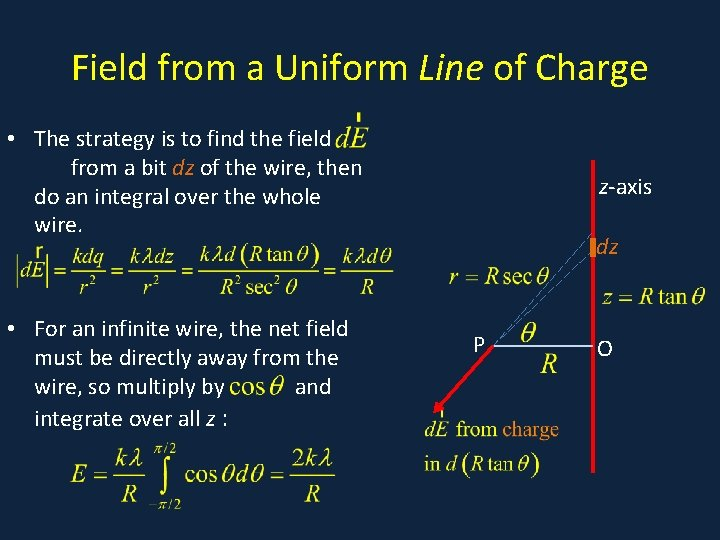Field from a Uniform Line of Charge • The strategy is to find the field • q z from a bit dz of the wire, then do an integral over the whole wire. • For an infinite wire, the net field must be directly away from the wire, so multiply by and integrate over all z : z-axis dz P O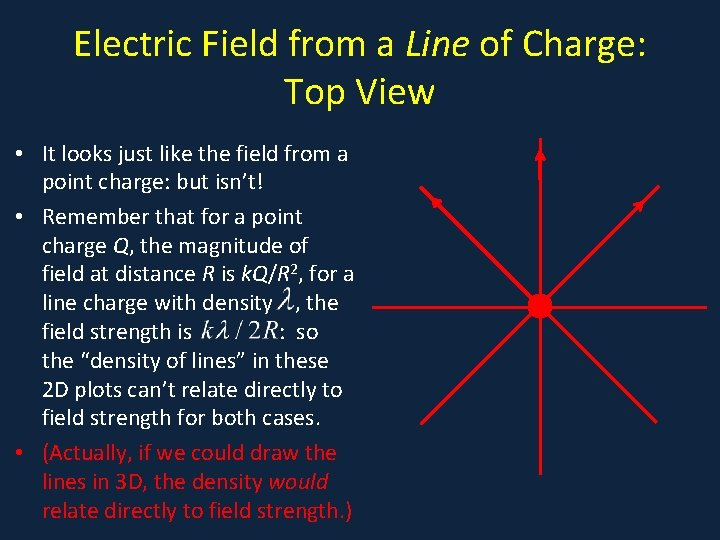Electric Field from a Line of Charge: Top View • It looks just like the field from a • a point charge: but isn’t! • Remember that for a point charge Q, the magnitude of field at distance R is k. Q/R 2, for a line charge with density , the field strength is : so the “density of lines” in these 2 D plots can’t relate directly to field strength for both cases. • (Actually, if we could draw the lines in 3 D, the density would relate directly to field strength. )Electric Field from a Plane of Charge: Top View Note: if you can’t follow this, it doesn’t matter! • Imagine now we have a uniformly charged plane: we make it up of many parallel wires, each charge density , each perpendicular to the page, with n wires/meter. • Remembering the field strength from a single wire is , and in dy there are ndy wires, the field strength at P from the charge in dy is: • Each red dot is a cross section of a wire perpendicular to the page. a dy P ONote: the next slide is all you need to know—and it’s simple! Electric Field from a Plane of Charge: Top View • We’ve shown the field strength at P from the charged lines in dy is • a • This has component in the OP direction dy The total field is given by integrating, P where the plane charge density Coulombs/m 2 , and • Notice the field strength is constant! O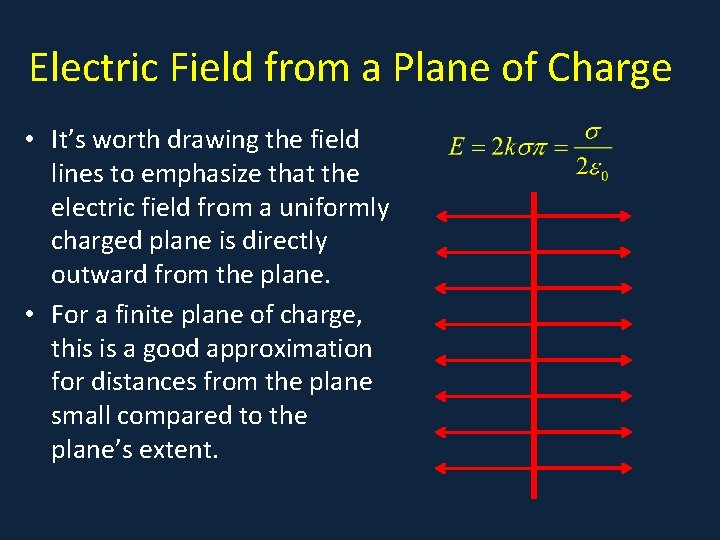Electric Field from a Plane of Charge • It’s worth drawing the field lines to emphasize that the • a electric field from a uniformly charged plane is directly outward from the plane. • For a finite plane of charge, this is a good approximation for distances from the plane small compared to the plane’s extent.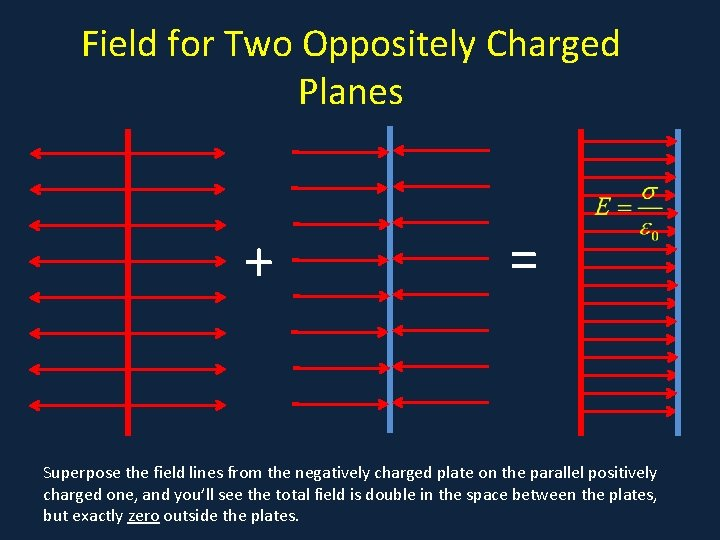Field for Two Oppositely Charged Planes • a + = Superpose the field lines from the negatively charged plate on the parallel positively charged one, and you’ll see the total field is double in the space between the plates, but exactly zero outside the plates.